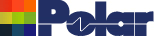Loss Tangent Goal Seek Application Note AP8193 Using the Si9000 Loss Tangent Goal Seek (Applies from Si9000e v16.05 onwards) Measuring insertion loss yields the total losses of a transmission line, but sometimes it is useful to further process that information and deduce the contribution of copper losses and dielectric losses to the overall loss figure. The Si9000e simplifies the complexity of the process of estimating dielectric loss by allowing you to: enter the total measured attenuation calculate an estimate of copper losses from cross section data remove the copper loss from the total attenuation to leave the losses from the substrate alone. This figure can then be processed to provide a useful estimate of the dielectric loss tangent for the substrate material. This application note describes the sequence of steps to goal seek for loss tangent. Surface microstrip example Select Surface Microstrip 1B structure with the default parameters: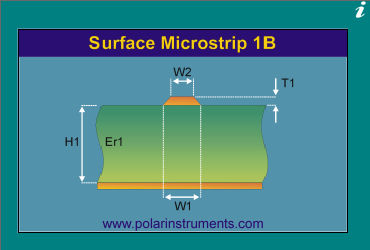From the Frequency Dependent tab select the Loss Tangent Goal Seek option.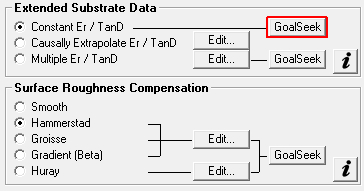The Loss Tangent Goal Seek dialog is displayed.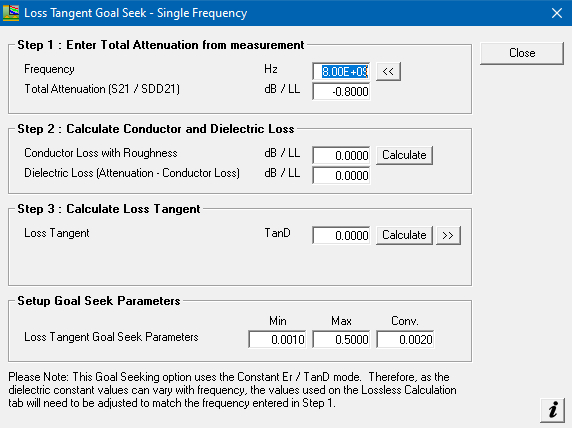Step 1 – Enter the Total Attenuation Under Step 1 enter the Total Attenuation for the point of interest, the frequency and the loss per length of line –dB / LL. The length of line will be the value entered into the Length of Line (LL) parameter on the main frequency dependent tab, so could be a 1000 mils for dB/inch or 10mm for dB/cm.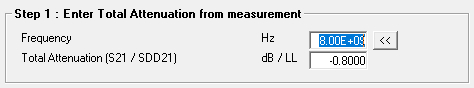In the case of the Surface Microstrip 1B structure default for LL is 1000 mils so dB/inch. In this example the Total Attenuation at 8GHz = –0.8 dB/inch Step 2 – Calculate the Conductor and Dielectric Loss Under Step 2, Calculate the Conductor and Dielectric Loss. This will take the parameters for the current selected structure (Surface Microstrip 1B) and calculate the conductor loss at 8GHz. It will then take the calculated conductor loss from the total attenuation entered in Step 1 to calculate the remaining dielectric loss.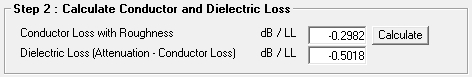In this case the conductor loss is -0.2981 dB/inch so the remaining dielectric loss is -0.5019 dB/inch Step 3 Calculate Loss Tangent The Step 3 Calculate Loss Tangent option will allow the Si9 to calculate the Loss Tangent (TanD) required to achieve a dielectric loss of -0.5019 dB/inch. Using the Goal Seek Parameters to limit the min / max range of TanD the Si9 will now sweep the range of TanD values until a suitable TanD is calculated to achieve a dielectric loss of –0.5019 dB/inch. Notice the progress of the calculation is updated on the dialog.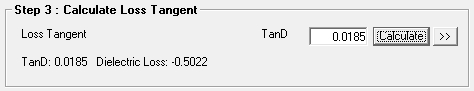The result is that the Loss Tangent (TanD) of 0.0185 is required to achieve a dielectric loss ~ –0.5019 dB/inch – the convergence value is used to give the target dielectric loss a tolerance. To verify, copy the TanD value of 0.0185, close the Loss Tangent Goal Seek dialog, enter it into the TanD field on the frequency dependent tab. Click Calculate to display the Loss v Frequency graph.Query the Conductor Loss with Roughness, Dielectric Loss and Attenuation with Roughness curve data points at 8GHz – notice the Attenuation with Roughness (total attenuation) is now ~ 0.8 dB/inch as specified in the Goal Seek dialog. If the total attenuation value entered in Step 1 of the Loss Tangent Goal Seek option is unachievable the Si9000 displays the message alert below: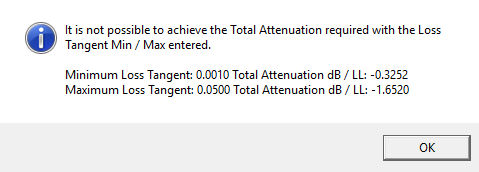The minimum and maximum Loss Tangent values as specified in the Setup Goal Seek Parameters are displayed along with the calculated Total Attenuation. If you are not already using Si9000e v16.05 or above please contact your local Polar Sales Office for more information.